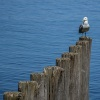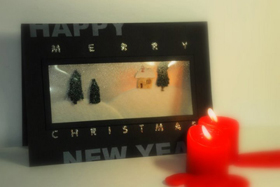#### You may also like### Even Up

Consider all of the five digit numbers which we can form using only the digits 2, 4, 6 and 8. If these numbers are arranged in ascending order, what is the 512th number?### Farey Sequences

There are lots of ideas to explore in these sequences of ordered fractions.### Writ Large

Suppose you had to begin the never ending task of writing out the natural numbers: 1, 2, 3, 4, 5.... and so on. What would be the 1000th digit you would write down.

# Greetings

##### Age 11 to 14 Challenge Level:
There are 30 students in a class and it is found that in any subset of 4 students from the class each student has exchanged Christmas cards with the other three. Show that some students have exchanged cards with all the other students in the class. How many such students are there?